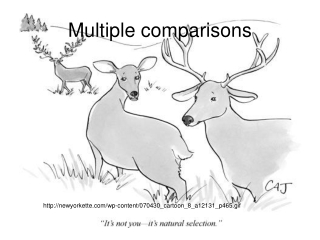DownloadDownload PresentationMultiple comparisons

# Multiple comparisons

Download Presentation## Multiple comparisons

- - - - - - - - - - - - - - - - - - - - - - - - - - - E N D - - - - - - - - - - - - - - - - - - - - - - - - - - -
##### Presentation Transcript

1. Multiple comparisons http://newyorkette.com/wp-content/070430_cartoon_8_a12131_p465.gif

2. How do we decide which means are truly different from one another? • ANOVAs only test the null hypothesis that the treatment means were all sampled from the same distribution…

3. Two general approaches • A posteriori comparisons: unplanned, after the fact. • A priori comparisons: planned, before the fact.

4. Effects of early snowmelt on alpine plant growth Three treatment groups and 4 replicates per treatment: Unmanipulated Heated with permanent solar-powered heating coils that melt spring snow pack earlier in the year than normal Controls, fitted with heating coils that are never activated

5. Data

6. ANOVA table for one-way layout P=tail of F-distribution with (a-1) and a(n-1) degrees of freedom

7. A posteriori comparisons • We will use Tukey’s “honestly significant differences” (HSD) • It controls for the fact that we are carrying out many simultaneous comparisons • the P-value is adjusted downward for each individual test to achieve an experiment-wise error rate of α=0.05

8. The HSD Where: q= is the value from a statistical table of the studentized range distribution n = sample size

9. The HSD 2.25, NS 3.25, P<0.05 1, NS So we find significant differences between the control and the heated and no significance between unmanipulated vs. control, or unmanipulated vs. heated…

10. Dunnett’s test For each treatment vs. control pair, CV= d(m,df) SEt; Where m (number of treatments) includes the control and d is found in tables for Dunnett’s test. Use Dunnett’s test by comparing treatment furthest from control first, then next furthest from control, etc.

11. Dunnett’s test d-critical(m=3,df=9)=2.61

12. But… • Occasionally posterior tests may indicate that none of the pairs of means are significantly different from one another, even if the overall F-ratio led to reject the null-hypothesis! • This inconsistence results because the pairwise test are not as powerful as the overall F-ratio itself.

13. A priori (planned) comparisons • They are more specific • Usually they are more powerful • It forces you to think clearly about which particular treatment differences are of interest

14. A priori (planned) comparisons • The idea is to establish contrasts, or specified comparisons between particular sets of means that test specific hypothesis • These test must be orthogonal or independent of one another • They should represent a mathematical partitioning of the among group sum of squares

15. To create a contrast • Assign an number (positive, negative or 0) to each treatment group • The sum of the coefficients for a particular contrast must equal 0 (zero) • Groups of means that are to be averaged together are assigned the same coefficient • Means that are not included in the comparison of a particular contrast are assigned a coefficient of 0

16. Contrast I (Heated vs. Non-Heated) F-ratio= 20.16/2.17=9.2934 F-critical1,9 =5.12 With 1 df

17. Contrast I (Heated vs. Non-Heated)using formula for non equal samples F-ratio= 20.16/2.17=9.2934 F-critical1,9 =5.12 With 1 df More information on Sokal and Rohlf (2000) Biometry

18. Contrast II (Control vs. Unmanipulated) F-ratio= 2/2.17=0.9217 F-critical1,9 =5.12 With 1 df

19. In order to create additional orthogonal contrasts • If there are a treatment groups, at most there can be (a-1) orthogonal contrasts created (although there are many possible sets of such orthogonal contrasts). • All of the pair-wise cross products must sum to zero. In other words, a pair of contrast Q and R is independent if the sum of the products of their coefficients CQi and CRi equals zero.

20. A priori contrasts